# Solution in Presence of Perfectly Conducting Wall

Suppose that the plasma occupies the region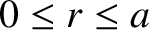, where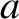is the plasma minor radius. It follows thatfor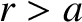. Let the plasma be surrounded by a concentric, rigid, radially-thin, perfectly conducting wall of radius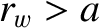. (In most circumstances, the wall represents the metallic vacuum vessel that surrounds the plasma.) An appropriate physical solution of the cylindrical tearing mode equation, (3.60), takes the separable form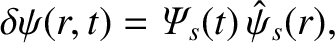(3.63)

where the real function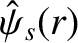is a solution of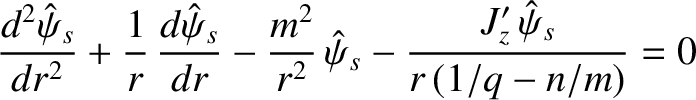(3.64)

that satisfies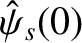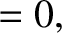(3.65)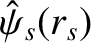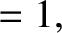(3.66)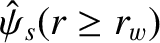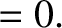(3.67)

Equation (3.65) ensures that the perturbed magnetic field associated with the tearing mode remains finite at the magnetic axis (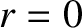), whereas Equation (3.67) represents the physical constraint that the perturbed magnetic field cannot penetrate a perfectly conducting wall.

Let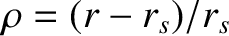. The solution of Equation (3.64) in the vicinity of the rational surface is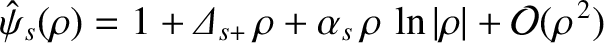(3.68)

for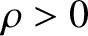, and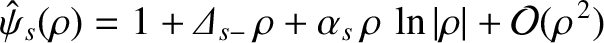(3.69)

for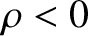. Here,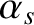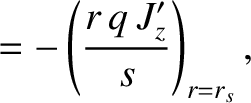(3.70)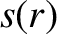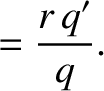(3.71)

Moreover, the real parameters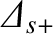and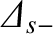are fully determined by Equation (3.64) and the boundary conditions (3.65)–(3.67). Note that, in general,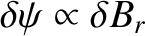is continuous across the rational surface (in accordance with Maxwell's equations), whereas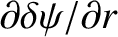is discontinuous. The discontinuity inimplies the presence of a radially-thin, helical current sheet at the rational surface. This current sheet is resolved in a thin resistive layer that, in principle, can only be described by employing the full set of neoclassical fluid equations, (2.370)–(2.374), rather than the reduced set of marginally-stable ideal-MHD equations, (2.375)–(2.380).

The value of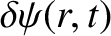at the rational surface,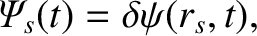(3.72)

is known as the reconnected magnetic flux . Note that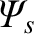is, in general, a complex quantity. The complex quantity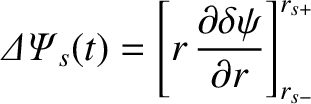(3.73)

parameterizes the amplitude and phase of the current sheet flowing (parallel to the equilibrium magnetic field) at the rational surface. Asymptotically matching the solutions in the so-called inner region (i.e., the region of the plasma in the immediate vicinity of the rational surface) and the so-called outer region (i.e., everywhere in the plasma other than the inner region) with the help of Equations (3.63), (3.68), (3.69), and (3.73), we obtain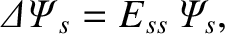(3.74)

where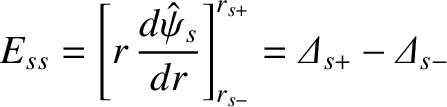(3.75)

is a real dimensionless quantity that is known as the tearing stability index .